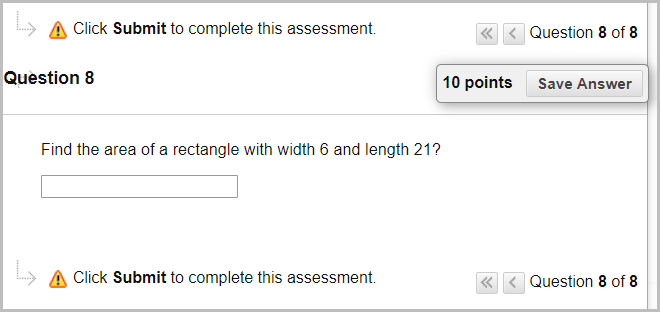# Learn.UQ Quizzes, Tests and Exams Online Workshop

## 12. Create Calculated Formula Question

### Overview

A Calculated Formula question contains a formula, the variables of which are different for each user. You specify the minimum and maximum value for each variable and answer sets are randomly generated.

### Example question

Find the area of a rectangle with width 6 and length 21?### Create a Calculated Formula Question

1. In your Learn.UQ course, from the Control Panel, select Course Tools > Tests, Surveys and Pools > Pools.
2. Click on the drop-down arrow next to Test Pool [your full name] then select Edit.
3. In your Pool, click on Create Question > Calculated Formula.
4. Enter "Find the area of a rectangle with width [W] and length [L]?" in the Question Text textbox.
5. Enter "W x L" in the Answer Formula textbox.

Note: You must use the symbols in the equation editor. No equal sign is required.

1. Click on the Next button.

### Define Variables

This section requires you to create variables and different Answer Sets so students receive random questions

1. For Variable Name, L, enter "10" in the Minimum Value textbox.
2. For Variable Name, L, enter "25" in the Maximum Value textbox.
3. For Variable Name, W, enter "3"in the Minimum Value textbox.
4. For Variable Name, W, enter "9" in the Maximum Value textbox.
5. For each variable, in Decimal Places, select "1" from the drop-down list.
6. For Calculate Answers to, select "1" from the drop-down list
7. Enter "20" in the Number of Answer Sets textbox.
8. Click on the Next button.

### Finalising Question

1. Click on the Submit button.